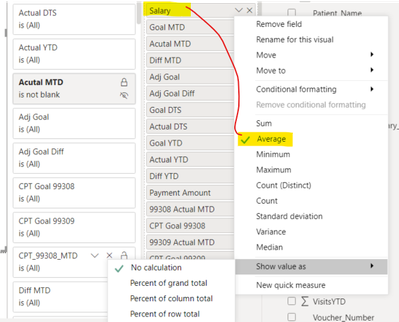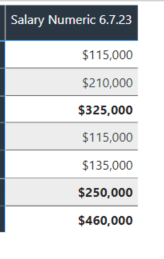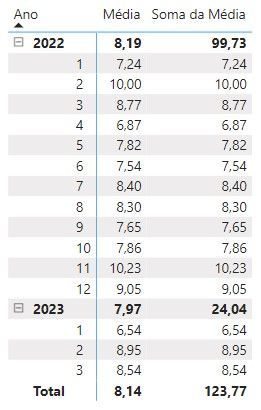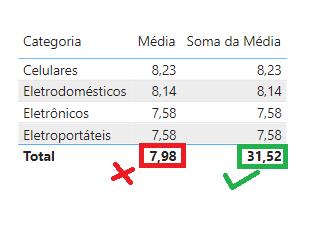cancel
Showing results for
Did you mean:Responsive Resident

## sum a total with a field that has an average

Hello and thanks in advance for helping me.

I have a table, and in my table I have a field for Average Salary, but I need the Average Salary to sum in the total.  Any suggestions on how to sum correctly?  Highlighted # below should be \$210,000, not \$105,425.Thank you 🙂10 REPLIES 10Responsive ResidentResponsive Resident

I'm basically using a Provider Name and Provider Salary.  Here is the formula for Provider Salary aka Salary Numeric 6.7.23.

Salary Numeric 6.7.23 = SUMX ( VALUES ( Dataset[Salary_Numeric] ), CALCULATE ( AVERAGE ( Dataset[Salary_Numeric] ) ) )

Grand Total is not working.

Can you provide examples on how I can get the formula to work?

Thank you 🙂Resolver I

I needed a sample of your data. But see if it helps

``````Salary Numeric 6.7.23 =
SUMX (
VALUES ( Dataset[Provider Name] ),
CALCULATE ( AVERAGE ( Dataset[Salary_Numeric] ) )
)``````Responsive Resident

Thank you for the reply.  The previously formula was not 100% accurate, although, I feel like you are on the right path.  The "Salaray Numeric 6.7.23" makes rthe row total correct, however, the Grand total is way off.  The newest formula you sent me also sums the row total correctly, and the Grand Total is only about half off the correct amount (When all Providers are selected).  Here is the last foirmula you sent me, which I feel is close: Salary Numeric 6.7.23 = SUMX ( VALUES ( Dataset[Provider Name] ), CALCULATE ( AVERAGE ( Dataset[Salary_Numeric] ) ) ).  Is it possible to create a formual that substitutes "Salary Numeric" with "Salary Numeric 6.7.23"?  It wont let substitute in the last formula you sent.  I'm assuming because it is a calculated measure, i dont know?  Thank you in advance!Resolver I

Seria bom que enviasse uma amostra dos seus dados. Pode ser fictícios.Responsive Resident

@VilmarSch That worked for the row totals using this formula ↓

Salary Numeric 6.7.23 = SUMX ( VALUES ( rpt_Reconciliation[Salary_Numeric] ), CALCULATE ( AVERAGE ( rpt_Reconciliation[Salary_Numeric] ) ) )

But now, the Grand Total is off.  Any suggestions on how to fix?  Thank you!Resolver I
``````Soma da Média =
VAR TabelaResumida =
SUMMARIZECOLUMNS ( dCalendar[Ano], dCalendar[MesId] )
VAR MediasMensais =
SUMX ( TabelaResumida, CALCULATE ( AVERAGE ( fVendas[Qtd] ) ) )
RETURN
MediasMensais``````Responsive Resident

@VilmarSch using the "Salary Numeric 6.7.23" formual I commented earlier.  Can you help me plug in the fields and caluclations that you referred to me on your last comment?Resolver IResolver I

Use medidas

Exemplo:

``````Soma da Média =
SUMX ( VALUES ( dProduto[Categoria] ), CALCULATE ( AVERAGE ( fVendas[Qtd] ) ) )``````